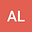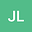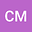Reconstructing a Rotor from Initial and Final Frames using Characteristic Multivectors: with applications in Orthogonal Transformations: Version 2
•••Charalampos Matsantonis
University of Cambridge
Author Profile## Abstract

If an initial frame of vectors $\{e_i\}$ is related to a final frame of vectors $\{f_i\}$ by, in geometric algebra (GA) terms, a {\it rotor}, or in linear algebra terms, an {\it orthogonal transformation}, we often want to find this rotor given the initial and final sets of vectors. One very common example is finding a rotor or $4\times 4$ orthogonal matrix representing rotation and translation, given knowledge of initial and transformed points. In this paper we discuss methods in the literature for recovering such rotors and then outline a GA method which generalises to cases of any signature and any dimension, and which is not restricted to orthonormal sets of vectors. The proof of this technique is both concise and elegant and uses the concept of {\it characteristic multivectors} as discussed in the book by Hestenes \& Sobczyk, which contains a treatment of linear algebra using geometric algebra. Expressing orthogonal transformations as rotors, enables us to create {\it fractional transformations} and we discuss this for some classic transforms. In real applications, our initial and/or final sets of vectors will be noisy. We show how to use the characteristic multivector method to find a ‘best fit’ rotor between these sets and compare our results with other methods.
30 Jan 2022Submitted to Mathematical Methods in the Applied Sciences
01 Feb 2022Submission Checks Completed
01 Feb 2022Assigned to Editor
07 Feb 2022Reviewer(s) Assigned
03 Jun 2022Review(s) Completed, Editorial Evaluation Pending
04 Jun 2022Editorial Decision: Revise Major